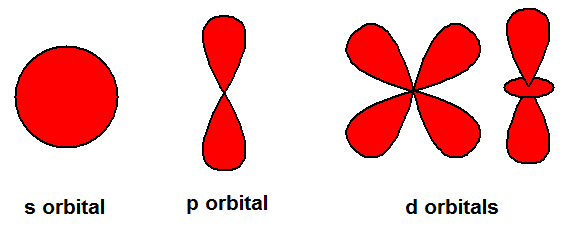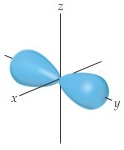# Problem: You may want to reference (Pages 232 - 235) Section 6.6 while completing this problem.The contour representation of one of the orbitals for the n = 3 shell of a hydrogen atom is shown in the figure below.In which of the following ways would you modify this sketch if the value of the magnetic quantum number, m𝓁, were to change?(i) It would be drawn larger(ii) The number of lobes would change(iii) The lobes of the orbital would point in a different direction(iv) There would be no change in the sketch

###### FREE Expert Solution

Recall the different shapes of the s, p, and d orbitals.The m values (for each ℓ) represent an orbital for the subshell:

s subshell → 1 orbital
p subshell → 3 orbitals
d subshell → 5 orbitals

Possible set of quantum #'s for n = 3:

• ℓ = 0 to n - 1
95% (205 ratings)###### Problem Details

You may want to reference (Pages 232 - 235) Section 6.6 while completing this problem.

The contour representation of one of the orbitals for the n = 3 shell of a hydrogen atom is shown in the figure below.In which of the following ways would you modify this sketch if the value of the magnetic quantum number, m𝓁, were to change?
(i) It would be drawn larger
(ii) The number of lobes would change
(iii) The lobes of the orbital would point in a different direction
(iv) There would be no change in the sketch

Frequently Asked Questions

What scientific concept do you need to know in order to solve this problem?

Our tutors have indicated that to solve this problem you will need to apply the Quantum Numbers: Magnetic Quantum Number concept. You can view video lessons to learn Quantum Numbers: Magnetic Quantum Number. Or if you need more Quantum Numbers: Magnetic Quantum Number practice, you can also practice Quantum Numbers: Magnetic Quantum Number practice problems.

What professor is this problem relevant for?

Based on our data, we think this problem is relevant for Professor Atwood's class at UB.